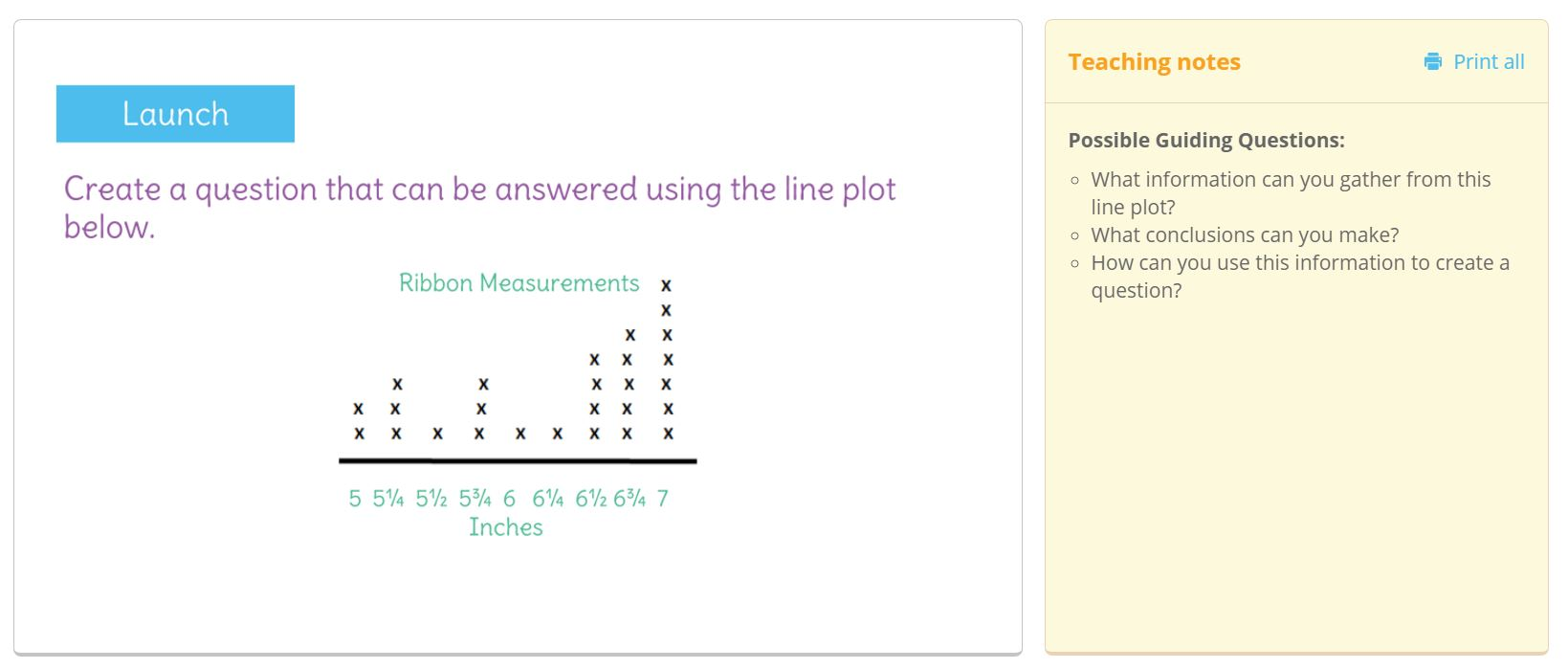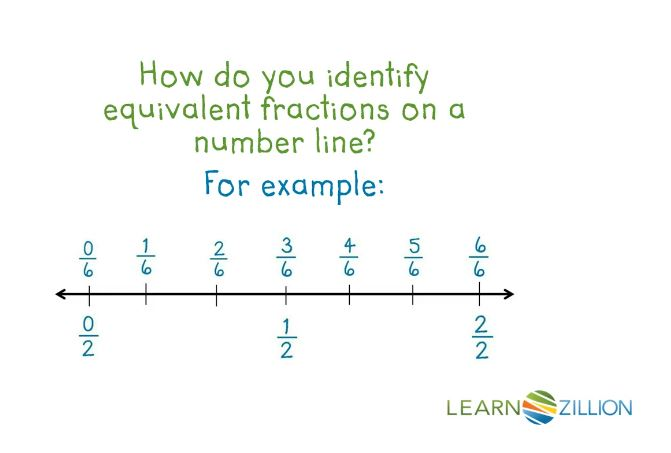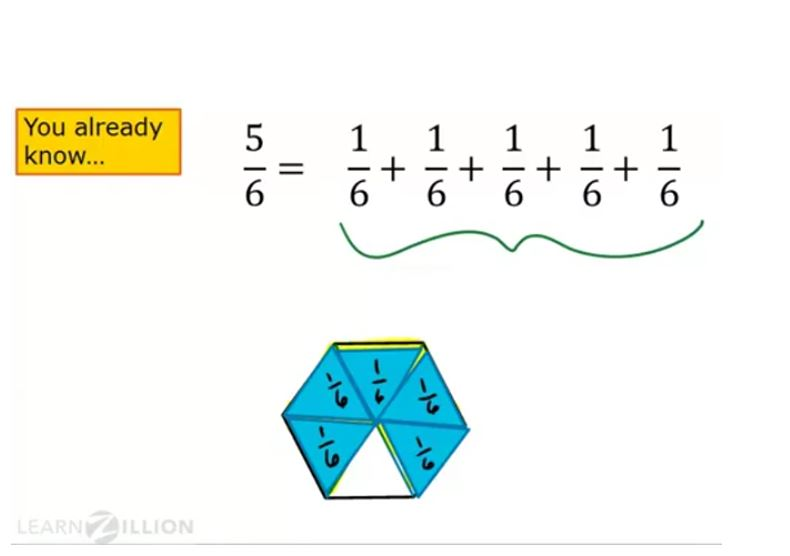# Looking at Line Plots in 4th Grade

In 3rd grade, students measured objects to the nearest half and quarter of an inch and displayed that data on a line plot. In standard MAFS.4.MD.2.4, 4th graders extend that learning to include displaying measurements to nearest half, quarter, and eighth of a unit on a line plot. They must also solve problems involving addition and subtraction of the fractions to interpret data from a line plot.

4th graders are required to synthesize a lot of prior learning: creating and interpreting line plots, measuring to nearest fraction of a unit, displaying fractional amounts on a number line/ordering fractions, and addition and subtraction of fractions (note that our FSA Item Specifications state that addition and subtraction are limited to like denominators). Students may need support with any one of these concepts; the resources below can be used to access their prior knowledge or to scaffold instruction.

“Magnify an Inch” – blog post with instructional ideas for teaching students about fractional parts of an inch; can easily be extended to include eighths“Measuring Length with Fractions” – blog post with lesson ideas for measuring objects to the nearest half and quarter of an inch; can also easily be extended to include eighthsLearnZillion Lesson: “Measure and Represent Objects to the Nearest Whole, Half, and Quarter Inch Using a Ruler and Line Plot” – lesson ideas to access students’ prior knowledge about measuring to nearest faction of a unit, and displaying and interpreting measurement data on a line plotLearnZillion Video- “Identify Equivalent Fractions on a Number Line” – video that can be used to reteach representing fractions on a number line, as well as using a number line to find equivalent fractions; students may need reteach on these skills in order to create the horizontal axis of their line plot to display measurement data to the nearest fraction of a unitLearnZillion Video: “Add and Subtract Fractions with Like Denominators” – video to revisit why only the numerators are added or subtracted when adding or subtracting with like denominators